+

# Valuing Different Costs

Author: Sophia Tutorial
##### Description:

Calculate the cost of debt, preferred stock, or common equity.

(more)### Developing Effective Teams

*No strings attached. This college course is 100% free and is worth 1 semester credit.

37 Sophia partners guarantee credit transfer.

299 Institutions have accepted or given pre-approval for credit transfer.

* The American Council on Education's College Credit Recommendation Service (ACE Credit®) has evaluated and recommended college credit for 32 of Sophia’s online courses. Many different colleges and universities consider ACE CREDIT recommendations in determining the applicability to their course and degree programs.

Tutorial

what's covered
In this lesson, you will learn about the cost of debt, preferred stock, or common equity. Specifically, this lesson will cover:
1. The Cost of Debt
2. The Cost of Preferred Stock
3. The Cost of Common Equity
4. The Cost of Retained Earnings
5. The Cost of New Common Stock
1. Direct Costs
2. Indirect Costs

## 1. The Cost of Debt

When financing a new project, business, or operation, organizations can utilize both equity and debt to create a balanced weighted average cost of capital. One of the primary differences between equity and debt pertains to risk, and the impact that risk has on the cost of capital. To understand the role of debt, and how the cost of debt impacts overall cost of capital, the weighted average cost of capital equation is a useful data point:

formula

Weighted Average Cost of Capital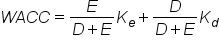When an organization borrows capital from outside lenders, the interest on these loans is called debt. The variables involved in a debt transaction are fairly simple:
• Kd: Cost of debt
• Rf: Risk-free rate
• t: Tax
• Credit risk rate: Risk premium
When these variables are plugged into a formula, it looks like this:

formula

Cost of Debt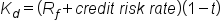The risk-free rate (or Rf) is externally determined from the general market, and is described as the overall cost incurred due to the time value of money with no risk whatsoever involved. This is usually derived from a government treasury bond, as it is the investment asset in most markets with the lowest possible rate of risk. Tax rates are set externally as well, and are concrete.

The credit risk rate is therefore the point of negotiation, and where a risk premium is attached to the debt instrument to compensate the investor in regards to a return (for the risk taken). As a general rule, the larger the debt is, the higher the risk rate will be (as all other things being equal, a higher debt is harder to pay back). Debtors will also take into consideration the collateral available to the organization (i.e., a valuation of their assets).

big idea
Determining the cost of debt is quite a bit easier than determining the cost of equity. The inputs to a debt assessment are tangibly determined, and consistent across the life of the agreement. It is worth noting that debt is generally less risky from the investor’s point of view, as debt obligations are paid out before equity obligations if a business goes bankrupt.

term to know

Weighted Average Cost of Capital (WACC)
A calculation of the various required returns on a project, which ultimately determines the profitability required to break even.

## 2. The Cost of Preferred Stock

Preferred stock is an equity security with properties of both an equity and a debt instrument. It is generally considered a hybrid instrument. Preferred stock represents some degree of ownership in a company, but usually doesn’t come with the same voting rights. In the event of liquidation, preferred shareholders are paid off before the common shareholder, but after debt holders. Preferred stock may also be callable or convertible, meaning that the company has the option to purchase the shares from shareholders at anytime for any reason – usually for a premium – or convert the shares to common stock. Similar to bonds, preferred stocks are rated by the major credit rating companies. Some people consider preferred stock to be more like debt than equity.

Because preferred stock carries a differing amount of risk than other types of securities, we must calculate its asset specific cost of capital to work into our overall weighted average cost of capital. Similar to debt, this can be a relatively simple process since we can observe values needed as inputs in the market. With preferred shares, investors are usually guaranteed a fixed dividend forever. This is different than common stock, which has variable dividends that are never guaranteed. If the preferred dividend is known and fixed, we can use the following equation to calculate the cost of capital for preferred stock.

formula

Cost of Preferred Stock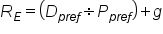This says that the cost of preferred stock is equal to the preferred dividend divided by the preferred stock price, plus the forecasted growth rate. Typically, the dividend is described as a percentage of a predetermined fixed amount, such as par value. These amounts may change based on negotiations, or by virtue of a benchmarked interest rate index.

try it
Question: Suppose the preferred dividend was \$10, the preferred stock price was \$100, and the forecasted growth rate is 3%. What is the cost of preferred stock?

Answer: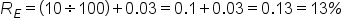term to know

Hybrid Instrument
A broad group of securities that pay a predictable (fixed or floating) rate of return or dividend until a certain date, at which point the holder has a number of options including converting the securities into the underlying share.

## 3. The Cost of Common Equity

As a funding source, debt and equity are priced quite differently. While debt relies heavily on default premiums being applied to a risk-free bond rate of interest (the premium usually dependent on the size of the debt compared to the size of the firm), equity isn’t quite as well-defined. As a result, the cost of common equity is often inferred through assessing and comparing it to similar risk profiles, and trying to decipher the firm’s relative sensitivity to systematic (market) risk.

One common tool used in assessing the cost of common equity is the capital asset pricing model (CAPM), which can be described via the following formula:

formula

Capital Asset Pricing Model (CAPM)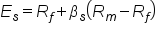What this formula is saying is essentially that the cost of equity is equal to the risk-free rate of return plus a premium for the expected risk of investing in the organization’s equity. The variable are defined as follows:
• Es: Expected return for a security
• Rf: Expected risk-free return rate
• βs: Sensitivity to market risk
• Rm: Historical return of the stock market
• (Rm – Rf): Risk premium of market assets over risk-free assets
The cost of common equity is essentially the same thing as estimating the expected (or required) return to investors in the stock market. The idea is simply that the higher risk an organization is, the higher the corresponding return should be. As a result, there are countless stock price evaluation strategies and tactics one could use to estimate the cost of common equity from various perspectives. Two of these include the dividend discount model and the Fama-French three-factor model.

Other Tools to Value Equity Equation Description
Dividend Discount Model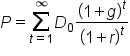In short, this theory states that a given stock is worth the sum of its future dividend payments, discounted to their present value. This is therefore a model of deriving the present value of future dividend payments, and can be calculated using the formula (assuming no end date).

In this calculation, P is the stock price, D is the dividend, g is the (constant) growth rate, r is the constant cost of equity, and t is time.

There are a few problems with this method, most notably that a steady and perpetual growth rate that is less than the cost of capital may not be reasonable. Similarly, this model is quite vulnerable to the growth rate. Also, not all stocks pay dividends.
Fama-French Three-Factor Model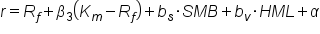This valuation model was derived by Eugene Fama and Kenneth French with the intention to include company size and price-to-book ratio with overall market risk.

You’ll recognize the first half of this equation as the simple CAPM calculation, while the second half includes SMB (small minus big market capitalization) and HML (high minus low book-to-market ratio) multiplied by coefficients (from linear regression).

While this all sounds a bit complicated, the basic premise is to offset the risk calculation with a stronger valuation for a firm’s assets and scale.

big idea
All and all, the valuation of common equity is always an estimation. As understanding the risk of a given organization within the stock market is intrinsically speculative, equity tends to be quite a bit more costly than debt. After all, when an organization goes bankrupt, it is the debtors who are reimbursed first, preferred stock next, and common stock last. This is the riskiest position of an investment.

## 4. The Cost of Retained Earnings

Retained earnings indicate the amount of capital remaining after profits or losses from net income are paid out to investors and shareholders via dividends. Retained earnings are reinvested back into the organization. When organizations create profits, these profits are not always entirely distributed to investors at the end of a reporting period. As a result, these retained earnings can essentially be viewed as a potential funding source for the organization.

No capital comes without costs, however, and the cost of this capital must be taken into account when calculating the weighted average cost of capital (WACC). Retained earnings are included in the WACC equation as equity, as dividends are a component of the return on capital to equity stakeholders, and thus will have a correspondingly weighted influence on the cost of equity.

Understanding the equation to determine the retention ratio adds some clarification for this point:

formula

Retention Ratio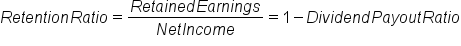The dividend payout ratio is a useful addition to the above equation, and is written as:

formula

Dividend Payout Ratio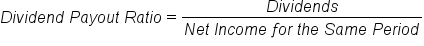The relationship between dividends and retained earnings is quite clear when it comes to recognizing the opportunity cost and thus the overall cost of this capital source.

hint
In the balance sheet, retained earnings is listed under equity, and is thus relative to the cost of equity.

## 5. The Cost of New Common Stock

When it comes to the cost of capital, common stock is one of a few options on the table for raising funding. From various debt instruments to preferred stock to common stock, larger organizations tend to diversify funding input to optimize their potential financial leverage. In order to understand the weighted average cost of capital (WACC) of all of these inputs, the cost of each source of debt and/or equity must be determined.

When it comes to issuing common stock, there are both direct and indirect costs to consider.

5a. Direct Costs
In terms of literal capital spent, the issuance of new common stock incurs a variety of capital costs both at the initial offering and throughout the process of managing this funding source over time:
• Legal fees for the distribution of business shares to the general public
• Increased accounting costs (if initial IPO) for management of a publicly traded firm
• Marketing costs for recruiting investors
• The time and effort of management
• Taxation (which differs region to region) pertaining to the distribution of equity
From a general perspective, the process of offering shares is skill-intensive; from management to legal to accounting, firms must hire and maintain a wide pool of talent to maintain this form of equity.

5b. Indirect Costs
In addition to the tangible capital costs involved, there are also a variety of indirect trade-offs that organizations must understand prior to pursuing this source of funding. Indirect costs include:
• Requirement to release financial information, including information that competitors may find valuable
• Compared to debt, it is less reliable as there may not be buyers interested at the desired price point
• Some loss of control, as the sale of shares is essentially the sale of organizational ownership
• Depending on the scale of shares available, the organization must consider takeover risks
As the issuance of publicly traded common stock is essentially the sale of the public auction of organizational equity, any and all considerations pertaining to control, ownership and legal implications should be considered as opportunity costs compared to other forms of funding.

summary
In this lesson, you learned in greater detail about the types of financing that factor into the weighted average cost of capital (WACC). You learned that the cost of debt involves several tangible variables, and is typically lower than the cost of equity because it carries less risk. The cost of capital rises as risk increases: the cost of preferred stock is higher than that of debt, but less than the cost of common equity, which is often derived through the capital asset pricing model. The cost of retained earnings is another capital cost that is included in the WACC equation as equity. Finally, you learned that the cost of new common stock includes direct costs associated with the transaction as well as indirect costs incurred by diluting organizational ownership.

Best of luck in your learning!

Source: THIS CONTENT HAS BEEN ADAPTED FROM LUMEN LEARNING'S “Valuing Different Costs” TUTORIAL.

Terms to Know
Hybrid Instrument

A broad group of securities that pay a predictable (fixed or floating) rate of return or dividend until a certain date, at which point the holder has a number of options including converting the securities into the underlying share.

Weighted Average Cost of Capital (WACC)

A calculation of the various required returns on a project, which ultimately determines the profitability required to break even.

Formulas to Know
Capital Asset Pricing Model (CAPM)Cost of DebtCost of Preferred StockDividend Payout RatioRetention RatioWeighted Average Cost of CapitalRating